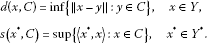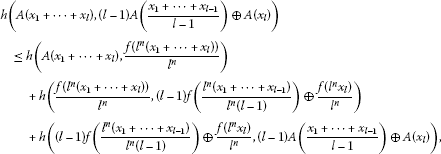# Hyers-Ulam stability of a generalized additive set-valued functional equation

## Abstract

In this paper, we define a generalized additive set-valued functional equation, which is related to the following generalized additive functional equation:

$f\left({x}_{1}+\cdots +{x}_{l}\right)=\left(l-1\right)f\left(\frac{{x}_{1}+\cdots +{x}_{l-1}}{l-1}\right)+f\left({x}_{l}\right)$

for a fixed integer l with $l>1$, and prove the Hyers-Ulam stability of the generalized additive set-valued functional equation.

MSC:39B52, 54C60, 91B44.

## 1 Introduction and preliminaries

The theory of set-valued functions has been much related to the control theory and the mathematical economics. After the pioneering papers written by Aumann  and Debreu , set-valued functions in Banach spaces have been developed in the last decades. We can refer to the papers by Arrow and Debreu , McKenzie , the monographs by Hindenbrand , Aubin and Frankowska , Castaing and Valadier , Klein and Thompson  and the survey by Hess .

The stability problem of functional equations originated from a question of Ulam  concerning the stability of group homomorphisms. Hyers  gave the first affirmative partial answer to the question of Ulam for Banach spaces. Hyers’ theorem was generalized by Aoki  for additive mappings and by Th.M. Rassias  for linear mappings by considering an unbounded Cauchy difference. A generalization of the Th.M. Rassias theorem was obtained by Găvruta  by replacing the unbounded Cauchy difference with a general control function in the spirit of Th.M. Rassias’ approach. The stability problems of several functional equations have been extensively investigated by a number of authors, and there are many interesting results concerning this problem (see ).

Let Y be a Banach space. We define the following:

${2}^{Y}$: the set of all subsets of Y;

${C}_{b}\left(Y\right)$: the set of all closed bounded subsets of Y;

${C}_{c}\left(Y\right)$: the set of all closed convex subsets of Y;

${C}_{cb}\left(Y\right)$: the set of all closed convex bounded subsets of Y.

On ${2}^{Y}$ we consider the addition and the scalar multiplication as follows:

$C+{C}^{\prime }=\left\{x+{x}^{\prime }:x\in C,{x}^{\prime }\in {C}^{\prime }\right\},\phantom{\rule{2em}{0ex}}\lambda C=\left\{\lambda x:x\in C\right\},$

where $C,{C}^{\prime }\in {2}^{Y}$ and $\lambda \in \mathbb{R}$. Further, if $C,{C}^{\prime }\in {C}_{c}\left(Y\right)$, then we denote $C\oplus {C}^{\prime }=\overline{C+{C}^{\prime }}$.

It is easy to check that

$\lambda C+\lambda {C}^{\prime }=\lambda \left(C+{C}^{\prime }\right),\phantom{\rule{2em}{0ex}}\left(\lambda +\mu \right)C\subseteq \lambda C+\mu C.$

Furthermore, when C is convex, we obtain $\left(\lambda +\mu \right)C=\lambda C+\mu C$ for all $\lambda ,\mu \in {\mathbb{R}}^{+}$.

For a given set $C\in {2}^{Y}$, the distance function $d\left(\cdot ,C\right)$ and the support function $s\left(\cdot ,C\right)$ are respectively defined byFor every pair $C,{C}^{\prime }\in {C}_{b}\left(Y\right)$, we define the Hausdorff distance between C and ${C}^{\prime }$ by

$h\left(C,{C}^{\prime }\right)=inf\left\{\lambda >0:C\subseteq {C}^{\prime }+\lambda {B}_{Y},{C}^{\prime }\subseteq C+\lambda {B}_{Y}\right\},$

where ${B}_{Y}$ is the closed unit ball in Y.

The following proposition reveals some properties of the Hausdorff distance.

Proposition 1.1 For every $C,{C}^{\prime },K,{K}^{\prime }\in {C}_{cb}\left(Y\right)$ and $\lambda >0$, the following properties hold:

1. (a)

$h\left(C\oplus {C}^{\prime },K\oplus {K}^{\prime }\right)\le h\left(C,K\right)+h\left({C}^{\prime },{K}^{\prime }\right)$;

2. (b)

$h\left(\lambda C,\lambda K\right)=\lambda h\left(C,K\right)$.

Let $\left({C}_{cb}\left(Y\right),\oplus ,h\right)$ be endowed with the Hausdorff distance h. Since Y is a Banach space, $\left({C}_{cb}\left(Y\right),\oplus ,h\right)$ is a complete metric semigroup (see ). Debreu  proved that $\left({C}_{cb}\left(Y\right),\oplus ,h\right)$ is isometrically embedded in a Banach space as follows.

Lemma 1.2 

Let $C\left({B}_{{Y}^{\ast }}\right)$ be the Banach space of continuous real-valued functions on ${B}_{{Y}^{\ast }}$ endowed with the uniform norm ${\parallel \cdot \parallel }_{u}$. Then the mapping $j:\left({C}_{cb}\left(Y\right),\oplus ,h\right)\to C\left({B}_{{Y}^{\ast }}\right)$, given by $j\left(A\right)=s\left(\cdot ,A\right)$, satisfies the following properties:

1. (a)

$j\left(A\oplus B\right)=j\left(A\right)+j\left(B\right)$;

2. (b)

$j\left(\lambda A\right)=\lambda j\left(A\right)$;

3. (c)

$h\left(A,B\right)={\parallel j\left(A\right)-j\left(B\right)\parallel }_{u}$;

4. (d)

$j\left({C}_{cb}\left(Y\right)\right)$ is closed in $C\left({B}_{{Y}^{\ast }}\right)$

for all $A,B\in {C}_{cb}\left(Y\right)$ and all $\lambda \ge 0$.

Let $f:\mathrm{\Omega }\to \left({C}_{cb}\left(Y\right),h\right)$ be a set-valued function from a complete finite measure space $\left(\mathrm{\Omega },\mathrm{\Sigma },\nu \right)$ into ${C}_{cb}\left(Y\right)$. Then f is Debreu integrable if the composition $j\circ f$ is Bochner integrable (see ). In this case, the Debreu integral of f in Ω is the unique element $\left(D\right){\int }_{\mathrm{\Omega }}f\phantom{\rule{0.2em}{0ex}}d\nu \in {C}_{cb}\left(Y\right)$ such that $j\left(\left(D\right){\int }_{\mathrm{\Omega }}f\phantom{\rule{0.2em}{0ex}}d\nu \right)$ is the Bochner integral of $j\circ f$. The set of Debreu integrable functions from Ω to ${C}_{cb}\left(Y\right)$ will be denoted by $D\left(\mathrm{\Omega },{C}_{cb}\left(Y\right)\right)$. Furthermore, on $D\left(\mathrm{\Omega },{C}_{cb}\left(Y\right)\right)$, we define $\left(f+g\right)\left(\omega \right)=f\left(\omega \right)\oplus g\left(\omega \right)$ for all $f,g\in D\left(\mathrm{\Omega },{C}_{cb}\left(Y\right)\right)$. Then we obtain that $\left(\left(\mathrm{\Omega },{C}_{cb}\left(Y\right)\right),+\right)$ is an abelian semigroup.

Set-valued functional equations have been extensively investigated by a number of authors, and there are many interesting results concerning this problem (see ).

In this paper, we define a generalized additive set-valued functional equation and prove the Hyers-Ulam stability of the generalized additive set-valued functional equation.

Throughout this paper, let X be a real vector space and Y be a Banach space.

## 2 Stability of a generalized additive set-valued functional equation

Definition 2.1 Let $f:X\to {C}_{cb}\left(Y\right)$. The generalized additive set-valued functional equation is defined by

$f\left({x}_{1}+\cdots +{x}_{l}\right)=\left(l-1\right)f\left(\frac{{x}_{1}+\cdots +{x}_{l-1}}{l-1}\right)\oplus f\left({x}_{l}\right)$
(1)

for all ${x}_{1},\dots ,{x}_{l}\in X$. Every solution of the generalized additive set-valued functional equation is called a generalized additive set-valued mapping.

Note that there are some examples in .

Theorem 2.2 Let $\phi :{X}^{l}\to \left[0,\mathrm{\infty }\right)$ be a function such that

$\stackrel{˜}{\phi }\left({x}_{1},\dots ,{x}_{l}\right):=\sum _{j=0}^{\mathrm{\infty }}\frac{1}{{l}^{j}}\phi \left({l}^{j}{x}_{1},\dots ,{l}^{j}{x}_{l}\right)<\mathrm{\infty }$
(2)

for all ${x}_{1},\dots ,{x}_{l}\in X$. Suppose that $f:X\to \left({C}_{cb}\left(Y\right),h\right)$ is a mapping satisfying

$h\left(f\left({x}_{1}+\cdots +{x}_{l}\right),\left(l-1\right)f\left(\frac{{x}_{1}+\cdots +{x}_{l-1}}{l-1}\right)\oplus f\left({x}_{l}\right)\right)\le \phi \left({x}_{1},\dots ,{x}_{l}\right)$
(3)

for all ${x}_{1},\dots ,{x}_{l}\in X$. Then there exists a unique generalized additive set-valued mapping $A:X\to \left({C}_{cb}\left(Y\right),h\right)$ such that

$h\left(f\left(x\right),A\left(x\right)\right)\le \frac{1}{l}\stackrel{˜}{\phi }\left(x,\dots ,x\right)$
(4)

for all $x\in X$.

Proof Let ${x}_{1}=\cdots ={x}_{l}=x$ in (3). Since $f\left(x\right)$ is convex, we get

$h\left(f\left(lx\right),lf\left(x\right)\right)\le \phi \left(x,\dots ,x\right),$
(5)

and if we replace x by ${l}^{n}x$, $n\in \mathbb{N}$ in (5), then we obtain

$h\left(f\left({l}^{n+1}x\right),lf\left({l}^{n}x\right)\right)\le \phi \left({l}^{n}x,\dots ,{l}^{n}x\right)$

and

$h\left(\frac{f\left({l}^{n+1}x\right)}{{l}^{n+1}},\frac{f\left({l}^{n}x\right)}{{l}^{n}}\right)\le \frac{1}{{l}^{n+1}}\phi \left({l}^{n}x,\dots ,{l}^{n}x\right).$

So,

$h\left(\frac{f\left({l}^{n}x\right)}{{l}^{n}},\frac{f\left({l}^{m}x\right)}{{l}^{m}}\right)\le \frac{1}{l}\sum _{j=m}^{n-1}\frac{1}{{l}^{j}}\phi \left({l}^{j}x,\dots ,{l}^{j}x\right)$
(6)

for all integers n, m with $n\ge m$. It follows from (2) and (6) that $\left\{\frac{f\left({l}^{n}x\right)}{{l}^{n}}\right\}$ is a Cauchy sequence in $\left({C}_{cb}\left(Y\right),h\right)$.

Let $A\left(x\right)={lim}_{n\to \mathrm{\infty }}\frac{f\left({l}^{n}x\right)}{{l}^{n}}$ for each $x\in X$. Then we claim that A is a generalized additive set-valued mapping. Note that

$h\left(\frac{f\left({l}^{n}\left({x}_{1}+\cdots +{x}_{l}\right)\right)}{{l}^{n}},\left(l-1\right)f\left(\frac{{l}^{n}\left({x}_{1}+\cdots +{x}_{l-1}\right)}{{l}^{n}\left(l-1\right)}\right)\oplus \frac{f\left({l}^{n}{x}_{l}\right)}{{l}^{n}}\right)\le \frac{1}{{l}^{n}}\phi \left({l}^{n}{x}_{1},\dots ,{l}^{n}{x}_{l}\right).$

Since $h\left(A\oplus B,C\oplus D\right)\le h\left(A,C\right)+h\left(B,D\right)$, we havewhich tends to zero as $n\to \mathrm{\infty }$. So, A is a generalized additive set-valued mapping. Letting $m=0$ and passing the limit $m\to \mathrm{\infty }$ in (6), we get the inequality (4).

Now, let $T:X\to \left({C}_{cb}\left(Y\right),h\right)$ be another generalized additive set-valued mapping satisfying (1) and (4). So,

$\begin{array}{rcl}h\left(A\left(x\right),T\left(x\right)\right)& =& \frac{1}{{l}^{n}}h\left(A\left({l}^{n}x\right),T\left({l}^{n}x\right)\right)\\ \le & \frac{1}{{l}^{n}}h\left(A\left({l}^{n}x\right),f\left({l}^{n}x\right)\right)+\frac{1}{{l}^{n}}h\left(T\left({l}^{n}x\right),f\left({l}^{n}x\right)\right)\\ \le & \frac{2}{{l}^{n+1}}\stackrel{˜}{\phi }\left({l}^{n}x,\dots ,{l}^{n}x\right),\end{array}$

which tends to zero as $n\to \mathrm{\infty }$ for all $x\in X$. So, we can conclude that $A\left(x\right)=T\left(x\right)$ for all $x\in X$, which proves the uniqueness of A, as desired. □

Corollary 2.3 Let $1>p>0$ and $\theta \ge 0$ be real numbers, and let X be a real normed space. Suppose that $f:X\to \left({C}_{cb}\left(Y\right),h\right)$ is a mapping satisfying

$h\left(f\left({x}_{1}+\cdots +{x}_{l}\right),\left(l-1\right)f\left(\frac{{x}_{1}+\cdots +{x}_{l-1}}{l-1}\right)\oplus f\left({x}_{l}\right)\right)\le \theta \sum _{j=1}^{l}{\parallel {x}_{j}\parallel }^{p}$
(7)

for all ${x}_{1},\dots ,{x}_{l}\in X$. Then there exists a unique generalized additive set-valued mapping $A:X\to Y$ satisfying

$h\left(f\left(x\right),A\left(x\right)\right)\le \frac{l\theta }{l-{l}^{p}}{\parallel x\parallel }^{p}$

for all $x\in X$.

Proof

The proof follows from Theorem 2.2 by taking

$\phi \left({x}_{1},\dots ,{x}_{l}\right):=\theta \sum _{j=1}^{l}{\parallel {x}_{j}\parallel }^{p}$

for all ${x}_{1},\dots ,{x}_{l}\in X$. □

Theorem 2.4 Let $\phi :{X}^{l}\to \left[0,\mathrm{\infty }\right)$ be a function such that

$\stackrel{˜}{\phi }\left({x}_{1},\dots ,{x}_{l}\right):=\sum _{j=1}^{\mathrm{\infty }}{l}^{j}\phi \left(\frac{{x}_{1}}{{l}^{j}},\dots ,\frac{{x}_{l}}{{2}^{j}}\right)<\mathrm{\infty }$

for all ${x}_{1},\dots ,{x}_{l}\in X$. Suppose that $f:X\to \left({C}_{cb}\left(Y\right),h\right)$ is a mapping satisfying (3). Then there exists a unique generalized additive set-valued mapping $A:X\to \left({C}_{cb}\left(Y\right),h\right)$ such that

$h\left(f\left(x\right),A\left(x\right)\right)\le \frac{1}{l}\stackrel{˜}{\phi }\left(x,\dots ,x\right)$

for all $x\in X$.

Proof It follows from (5) that

$h\left(f\left(x\right),lf\left(\frac{x}{l}\right)\right)\le \phi \left(\frac{x}{l},\dots ,\frac{x}{l}\right)$

for all $x\in X$.

The rest of the proof is similar to the proof of Theorem 2.2. □

Corollary 2.5 Let $p>1$ and $\theta \ge 0$ be real numbers, and let X be a real normed space. Suppose that $f:X\to \left({C}_{cb}\left(Y\right),h\right)$ is a mapping satisfying (7). Then there exists a unique generalized additive set-valued mapping $A:X\to Y$ satisfying

$h\left(f\left(x\right),A\left(x\right)\right)\le \frac{l\theta }{{l}^{p}-l}{\parallel x\parallel }^{p}$

for all $x\in X$.

Proof

The proof follows from Theorem 2.4 by taking

$\phi \left({x}_{1},\dots ,{x}_{l}\right):=\theta \sum _{j=1}^{l}{\parallel {x}_{j}\parallel }^{p}$

for all ${x}_{1},\dots ,{x}_{l}\in X$. □

## References

1. Aumann RJ: Integrals of set-valued functions. J. Math. Anal. Appl. 1965, 12: 1–12. 10.1016/0022-247X(65)90049-1

2. Debreu G: Integration of correspondences. II. Proceedings of Fifth Berkeley Symposium on Mathematical Statistics and Probability 1967, 351–372. part I

3. Arrow KJ, Debreu G: Existence of an equilibrium for a competitive economy. Econometrica 1954, 22: 265–290. 10.2307/1907353

4. McKenzie LW: On the existence of general equilibrium for a competitive market. Econometrica 1959, 27: 54–71. 10.2307/1907777

5. Hindenbrand W: Core and Equilibria of a Large Economy. Princeton University Press, Princeton; 1974.

6. Aubin JP, Frankow H: Set-Valued Analysis. Birkhäuser, Boston; 1990.

7. Castaing C, Valadier M Lect. Notes in Math. 580. In Convex Analysis and Measurable Multifunctions. Springer, Berlin; 1977.

8. Klein E, Thompson A: Theory of Correspondence. Wiley, New York; 1984.

9. Hess C: Set-valued integration and set-valued probability theory: an overview. In Handbook of Measure Theory. North-Holland, Amsterdam; 2002. vols. I, II

10. Ulam SM: Problems in Modern Mathematics. Wiley, New York; 1940. chapter VI, Science edn.

11. Hyers DH: On the stability of the linear functional equation. Proc. Natl. Acad. Sci. USA 1941, 27: 222–224. 10.1073/pnas.27.4.222

12. Aoki T: On the stability of the linear transformation in Banach spaces. J. Math. Soc. Jpn. 1950, 2: 64–66. 10.2969/jmsj/00210064

13. Rassias TM: On the stability of the linear mapping in Banach spaces. Proc. Am. Math. Soc. 1978, 72: 297–300. 10.1090/S0002-9939-1978-0507327-1

14. Gǎvruta P: A generalization of the Hyers-Ulam-Rassias stability of approximately additive mappings. J. Math. Anal. Appl. 1994, 184: 431–436. 10.1006/jmaa.1994.1211

15. Aczel J, Dhombres J: Functional Equations in Several Variables. Cambridge University Press, Cambridge; 1989.

16. Eshaghi Gordji M, Savadkouhi MB: Stability of a mixed type cubic-quartic functional equation in non-Archimedean spaces. Appl. Math. Lett. 2010, 23: 1198–1202. 10.1016/j.aml.2010.05.011

17. Hyers DH, Isac G, Rassias TM: Stability of Functional Equations in Several Variables. Birkhäuser, Basel; 1998.

18. Cascales T, Rodrigeuz J: Birkhoff integral for multi-valued functions. J. Math. Anal. Appl. 2004, 297: 540–560. 10.1016/j.jmaa.2004.03.026

19. Cardinali T, Nikodem K, Papalini F: Some results on stability and characterization of K -convexity of set-valued functions. Ann. Pol. Math. 1993, 58: 185–192.

20. Lu G, Park C: Hyers-Ulam stability of additive set-valued functional equations. Appl. Math. Lett. 2011, 24: 1312–1316. 10.1016/j.aml.2011.02.024

21. Nikodem K: On quadratic set-valued functions. Publ. Math. (Debr.) 1984, 30: 297–301.

22. Nikodem K: On Jensen’s functional equation for set-valued functions. Rad. Mat. 1987, 3: 23–33.

23. Nikodem K: Set-valued solutions of the Pexider functional equation. Funkc. Ekvacioj 1988, 31: 227–231.

24. Nikodem, K: K-Convex and K-Concave Set-Valued Functions. Zeszyty Naukowe 559, Lodz (1989)

25. Park C, O’Regan D, Saadati R: Stability of some set-valued functional equations. Appl. Math. Lett. 2011, 24: 1910–1914. 10.1016/j.aml.2011.05.017

26. Piao YJ:The existence and uniqueness of additive selection for $\left(\alpha ,\beta \right)\text{-}\left(\beta ,\alpha \right)$ type subadditive set-valued maps. J. Northeast Normal Univ. 2009, 41: 38–40.

27. Popa D:Additive selections of $\left(\alpha ,\beta \right)$-subadditive set-valued maps. Glas. Mat. 2001, 36(56):11–16.

28. Lee, K: Stability of functional equations related to set-valued functions. Preprint

## Acknowledgements

SYJ was supported by the 2012 Research Fund of University of Ulsan and had written this paper during visiting the Research Institute of Mathematics, Seoul National University. CP was supported by Basic Science Research Program through the National Research Foundation of Korea funded by the Ministry of Education, Science and Technology (NRF-2012R1A1A2004299).

## Author information

Authors

### Corresponding author

Correspondence to Young Cho.

### Competing interests

The authors declare that they have no competing interests.

### Authors’ contributions

All authors conceived of the study, participated in its design and coordination, drafted the manuscript, participated in the sequence alignment, and read and approved the final manuscript.

## Rights and permissions

Reprints and Permissions

Jang, S.Y., Park, C. & Cho, Y. Hyers-Ulam stability of a generalized additive set-valued functional equation. J Inequal Appl 2013, 101 (2013). https://doi.org/10.1186/1029-242X-2013-101

• Accepted:

• Published:

• DOI: https://doi.org/10.1186/1029-242X-2013-101

### Keywords

• Hyers-Ulam stability
• generalized additive set-valued functional equation
• closed and convex set
• cone